# The Surface Area of a Sphere Between Parallel Planes

Here’s a math problem that surprised me: Imagine a sphere of diameter d intersected by two parallel planes a distance h apart. What do you think the surface area of the section of the sphere between the planes is? You’re first thought might be that there’s not enough information. The area will depend upon what part of the sphere the planes intersect. If the planes cut out the middle third of the sphere, that will be a different surface area compared to if the planes cut out the left third or the right third.

That was my first thought, at least, and, as it turns out, it’s wrong. The area only depends on the distance between the planes, and the diameter of the sphere. It doesn’t matter where these planes are in relation to the sphere. As long as they both intersect the sphere, the surface area will equal πdh, where d is the diameter and h is the distance between the planes. Don’t believe me? Here’s the math to prove it:

First we begin with the formula for the surface area of a function rotated around the x axis from a to b. If you want an explanation of this equation, the section of my textbook that covers this is actually posted online [PDF] by the publishers (also note that this is problem 32 from that chapter).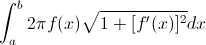Since we are finding the surface area of a sphere, we need the function for a circle, which we will rotate around the x axis, thus producing our sphere. If we can remember back to high school geometry, we’ll know that the equation for a sphere is x2+y2=r2. We now solve for y, and plug it in for f(x), and also find the derivative of f(x) and plug it in for f’(x):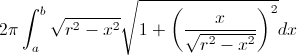We now simplify the integral: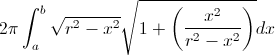f(x) is moved into the square root: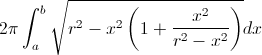We distribute: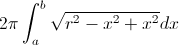More simplification: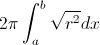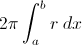We now integrate: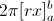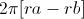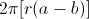b-a is simply the distance between the two planes, which we call h: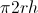2r is the diameter of the sphere, which we call d: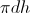There you have it. The surface area of a sphere between two parallel planes is equal to πdh. It doesn’t matter where these planes are in relation to the sphere. All that matters is that both planes intersect the sphere. Cool, huh? No? Ok, fine. Well, I thought it was interesting.